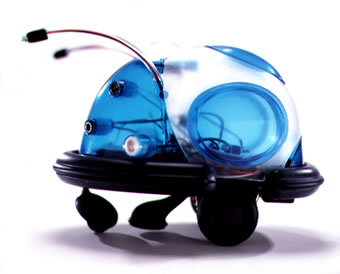# Stanley, Grand Challenge 2005

Beer bottle pass starts at 4.18

Show to 1.23

# One way robots think. Probability!

We will represent probabilities as the chance something might happen as P so

• P(I win the Lottery)=something very small. perhaps 1 in 100 million
• P(A coin I toss is heads)=roughly 50%, 50 times out of 100, 0.5

Of course winning the lottery has no effect on tossing a coin, but sometimes, events are linked....

# Bayes Theorem

Bayes Theorem is $P(G|O)=\frac{P(O|G)P(G)}{P(O)}$ Gives the probability of event G given observation O based on prior information (all the terms on the right of the =)

• Bayes Theorem has been used in robotics, medicine, psychology, neuroscience, statistics, ...

# Bayes Theorem example. Gender classification

If observation ($O$) is whether a person has a beard (B) and we wish to guess the gender ($G$) then

$P(G=male\,\,|\,\, O=beard)=\frac{P(O=beard\,\,|\,\,G=male)P(G=male)}{P(O=beard)}$ $P(G=female\,\,|\,\, O=beard)=\frac{P(O=beard\,\,|\,\,G=female)P(G=female)}{P(O=beard)}$

A little short cut, $P(O=beard)=0.5\left(P(O=beard\,\,|\,\,G=female)+P(O=beard\,\,|\,\,G=male)\right)$

# Bayes Theorem: Multiple observations example

If we make several observations then we can extend Bayes Theorem so

We want to determine the event `Gender=female', i.e. $G=F$

Let

• observation1 ($O_1$) give whether a person is wearing a dress (D)
• observation2 ($O_2$) say whether a person has long hair (L)

then $P(G=F\,|\, O_1=D\,\, \hbox{and}\,\, O_2 = L)\approx \frac{P(O_1=D\,\,|\,\,G=F)P(O_2=L\,|\,G=F)P(G=F)}{P(O_1=D)P(O_2=L)}$

# Why conditional probabilities are important

Through experience we as humans have learned a mechanism something like Bayesian priors to allow us to make gender judgements on

• wizards (such as Gandalf)
• cultural traditions (e.g. the Greek Evzones)
• drag persona such as Conchita Wurst
• cross dressers such as Grayson Perry

Is this how brains work and if so, should we make robots think like this?

# Some Robots from the University of Readinga)Real robots. b)Dave the funky shoulder monkey. c)Hasbro R2D2. d)Mech r/c robot.